# Point PropertyManager

The Point PropertyManager appears when you create a new reference point or edit an existing reference point.

When you select items, the SOLIDWORKS software attempts to select the appropriate point construction method. For example, if you select a face, SOLIDWORKS selects the Center of Face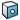construction method in the PropertyManager. You can always select a different type of point construction method.

To open this PropertyManager:

Click Point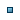(Reference Geometry toolbar) or Insert > Reference Geometry > Point.

## Selections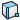Reference Entities Displays the selected entities used to create a reference point.
You can create reference points at the intersections of the following entities:
• An axis and a plane
• An axis and a surface, both planar and non-planar
• Two axes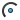Arc Center Creates a reference point at the center of the selected arc or circle.Center Of Face Creates a reference point at the center of mass of the selected face. You can select planar or non-planar faces.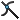Intersection Creates a reference point at the intersection of the two selected entities. You can select edges, curves, and sketch segments.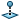Projection Creates a reference point from one entity projected onto another. Select two entities: the entity to project and the entity onto which to project. You can project points, endpoints of curves and sketch segments, and vertices of solids and surfaces onto planes and faces (planar or non-planar). The point is projected normal to the plane or face.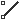On point Creates a reference point on a sketch point and on the end of a sketch section.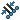Along curve distance or multiple reference point Creates a set of reference points along edges, curves, or sketch segments. Select the entity and create the reference points using these options:
 Enter the distance/percentage value according to distance Set a distance or percentage value to create the reference points. If the value is too large to create the specified number of reference points, a message warns you to set a smaller value. Distance Creates the number of reference points spaced at the distance you set. The first reference point is created at this distance from the endpoint, not on the endpoint. Percentage Creates the number of reference points spaced at the percentage you set. Percentage means percentage of the length of the selected entity. For example, select a 100mm-long entity. If you set the number of reference points to 5 and the percentage to 10, the 5 reference points are spaced 10 percent of the total entity length (or 10mm) apart from each other. Evenly Distribute Evenly distributes the number of reference points on the entity. If you edit the number of reference points, the reference points update their position relative to the starting endpoint.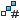Number of reference points Set the number of reference points to create along the selected entity. The reference points are created using the selected Distance, Percentage, or Evenly Distribute option.